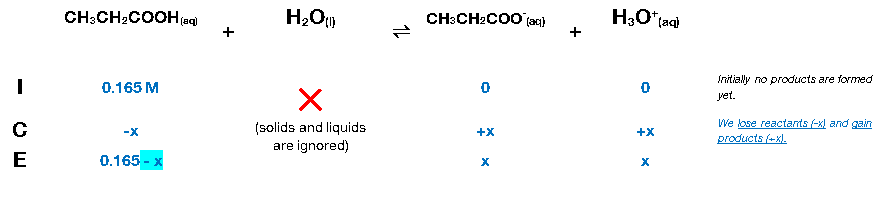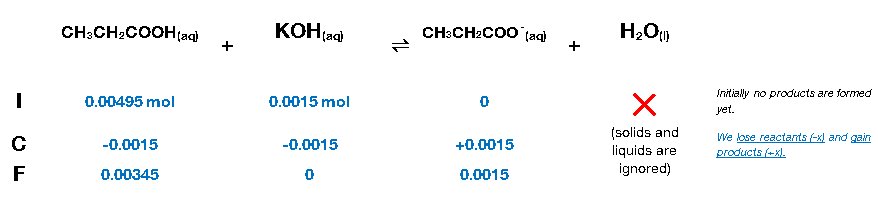# Problem: A 30.0 ml sample of 0.165 M propanoic acid is titrated with 0.300 M KOH.a. Calculate the pH at 0 mL of added base.b. Calculate the pH at 5 mL of added base.c. Calculate the pH at 10 mL of added base.d. Calculate the pH at the equivalent point.e. Calculate the pH at one-half of the equivalence point.f. Calculate the pH at 20 mL of added base.g. Calculate the pH at 25 mL of added base.

🤓 Based on our data, we think this question is relevant for Professor Nishida's class at WSU.

###### FREE Expert Solution

The dissociation of propanoic acid (CH3CH2COOH) is as follows:

CH3CH2COOH(aq) + H2O(l)  CH3CH2COO(aq) + H3O+(l);   Ka = 1.3x10-5

We can construct an ICE table. Remember that liquids are ignored in the ICE table.The Ka expression for CH3CH2COOH is:

$\overline{){{\mathbf{K}}}_{{\mathbf{a}}}{\mathbf{=}}\frac{\mathbf{reactants}}{\mathbf{products}}{\mathbf{=}}\frac{\mathbf{\left[}{\mathbf{CH}}_{\mathbf{3}}{\mathbf{CH}}_{\mathbf{3}}{\mathbf{COO}}^{\mathbf{-}}\mathbf{\right]}\mathbf{\left[}{\mathbf{H}}_{\mathbf{3}}{\mathbf{O}}^{\mathbf{+}}\mathbf{\right]}}{\left[{\mathrm{CH}}_{3}{\mathrm{CH}}_{2}\mathrm{COOH}\right]}}$

**Liquids are ignored in the Ka expression

Note that each concentration is raised by the stoichiometric coefficient: [CH3CH2COOH], [H3O+] and [CH3CH2COO] are raised to 1.

Plugging in the equilibrium concentrations from the ICE table into the Ka expression:

$\mathbf{1}\mathbf{.}\mathbf{3}\mathbf{×}{\mathbf{10}}^{\mathbf{-}\mathbf{5}}\mathbf{=}\frac{\mathbf{\left(}\mathbf{x}\mathbf{\right)}\mathbf{\left(}\mathbf{x}\mathbf{\right)}}{\left(0.165-x\right)}\phantom{\rule{0ex}{0ex}}\mathbf{1}\mathbf{.}\mathbf{3}\mathbf{×}{\mathbf{10}}^{\mathbf{-}\mathbf{5}}\mathbf{=}\frac{{\mathbf{x}}^{\mathbf{2}}}{\mathbf{0}\mathbf{.}\mathbf{165}\mathbf{-}\mathbf{x}}$

Now, we need to determine if we can remove (–x) from the equation. To do so, we need to determine the ratio of the initial concentration and Ka:

$\frac{{\mathbf{\left[}{\mathbf{HC}}_{\mathbf{3}}{\mathbf{H}}_{\mathbf{5}}{\mathbf{O}}_{\mathbf{3}}\mathbf{\right]}}_{\mathbf{initital}}}{{\mathbf{K}}_{\mathbf{a}}}\mathbf{=}\frac{\mathbf{0}\mathbf{.}\mathbf{165}}{\mathbf{1}\mathbf{.}\mathbf{3}\mathbf{×}{\mathbf{10}}^{\mathbf{-}\mathbf{5}}}\mathbf{=}\mathbf{>}\mathbf{>}\mathbf{>}\mathbf{500}$

Since the ratio is greater than 500, we can remove (–x) from the equation. the equation becomes:

x = 1.46×10-3

This means [H3O+] = [CH3CH2COO] = x = 1.76x10-3 M. From [H3O+], we can calculate for pH:

pH = 2.83→before KOH is added (0 mL)

b) After adding 5 mL KOH

Calculate the initial amounts of CH3CH2COOH and KOH in moles before the reaction happens.

Recall that molarity is given by:

molarity (volume) → moles

*convert volumes from mL to L → 1 mL = 10-3 L

30 mL of 0.165 M propanoic acid

moles CH3CH2COOH = 0.00495 mol

5 mL of 0.300 M KOH

moles KOH = 0.0015 mol

Construct an ICF Chart.After the reaction is complete the solution contains:

▪ 0.00345 mol CH3CH2COOH → weak acid

▪ 0.0015 mol CH3CH2COO-  conjugate base

whenever we have a conjugate base and a weak acid, we have a buffer

Since we have a buffer, we can calculate the pH of the solution using the Henderson-Hasselbalch equation:

pKa can be calculated from Ka:

*substituting in the Henderson-Hasselbalch equation:

Calculate pH:

*we can use moles of the conjugate base and the conjugate acid because we’re calculating the ratio

pH = 4.37→After adding KOH (5 mL)

c) After adding 10 mL KOH

Calculate the initial amounts of CH3CH2COOH and KOH in moles before the reaction happens.

Recall that molarity is given by:

molarity (volume) → moles

*convert volumes from mL to L → 1 mL = 10-3 L###### Problem Details

A 30.0 ml sample of 0.165 M propanoic acid is titrated with 0.300 M KOH.

a. Calculate the pH at 0 mL of added base.

b. Calculate the pH at 5 mL of added base.

c. Calculate the pH at 10 mL of added base.

d. Calculate the pH at the equivalent point.

e. Calculate the pH at one-half of the equivalence point.

f. Calculate the pH at 20 mL of added base.

g. Calculate the pH at 25 mL of added base.